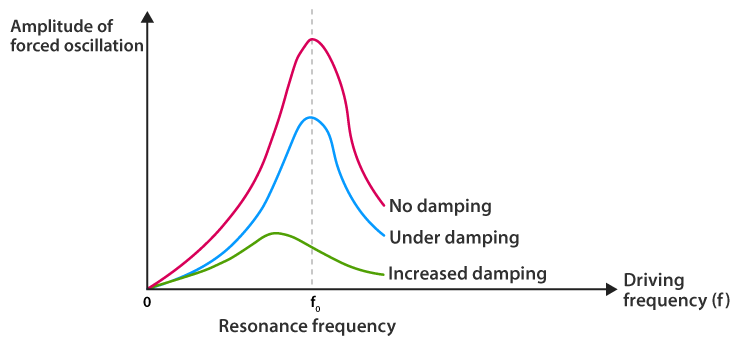Checkout JEE MAINS 2022 Question Paper Analysis : Checkout JEE MAINS 2022 Question Paper Analysis :

# Sharpness Of Resonance

## What is resonance?

The sharpness of resonance can be understood better by understanding resonance. Resonance is defined as the tendency of a system to vibrate with an increase in amplitude at the excitation of frequencies. Resonance frequency or resonant frequency is the maximum frequency at which the amplitude is relatively maximum. The Q factor is used to define the sharpness of the resonance.

## What is sharpness of resonance?

The sharpness of resonance is defined using the Q factor which explains how fast energy decay in an oscillating system. The sharpness of resonance depends upon:

• Damping: Effect due to which there is a reduction in amplitude of vibrations
• Amplitude: Maximum displacement of a point on a vibrating body which is measured from its equilibrium position.

The sharpness of resonance increases or decreases with an increase or decrease in damping and as the amplitude increases, the sharpness of resonance decreases.## What is Q factor?

Q factor or quality factor is a dimensionless parameter that is used to describe the underdamped resonator and characterizes the bandwidth and center frequency of the resonator.

The mathematical representation is:

Q = Estored/Elost per cycle

The Q factor of an RF resonant circuit is given as:

$$\begin{array}{l}Q=\frac{F_{0}}{F_{3dB}}\end{array}$$

## How does sharpness of resonance depend on damping?

The sharpness of resonance increases with an increase in damping and decreases with a decrease in damping.

## What is Q factor of coil?

Q factor for a coil is defined for a given frequency as the ratio of inductance L to the resistance R of a coil.

$$\begin{array}{l}Q=\frac{\omega L}{R}\end{array}$$

## What is Q factor of RLC circuit?

Q factor in a series circuit is:

$$\begin{array}{l}Q=\frac{1}{R}\sqrt{\frac{L}{C}}=\frac{\omega _{0}L}{R}=\frac{1}{\omega _{0}RC}\end{array}$$

Where,

R: resistance

L: inductance

C: capacitance

Q factor in a parallel circuit is:

$$\begin{array}{l}Q=R\sqrt{\frac{C}{L}}=\frac{R}{\omega _{0}L}={\omega _{0}RC}\end{array}$$

Where,

RLC are in parallel.

Related Physics Articles:

## Frequently Asked Questions – FAQs

### What is resonance?

Resonance is defined as the tendency of a system to vibrate with an increase in amplitude at the excitation of frequencies.

### Define resonant frequency.

Resonance frequency or resonant frequency is the maximum frequency at which the amplitude is relatively maximum.

### What is the sharpness of resonance?

The sharpness of resonance is defined using the Q factor which explains how fast energy decay in an oscillating system. The sharpness of resonance depends upon:
• Damping: Effect due to which there is a reduction in amplitude of vibrations
• Amplitude: Maximum displacement of a point on a vibrating body which is measured from its equilibrium position.

### What is the Q factor?

Q factor or quality factor is a dimensionless parameter that is used to describe the underdamped resonator and characterises the bandwidth and centre frequency of the resonator.

### What causes resonance to occur?

Resonance occurs when the matching vibrations of another object increase the amplitude of an object’s oscillations

Test your Knowledge on Sharpness Of Resonance